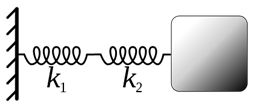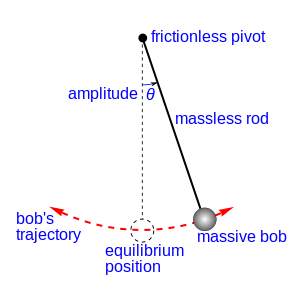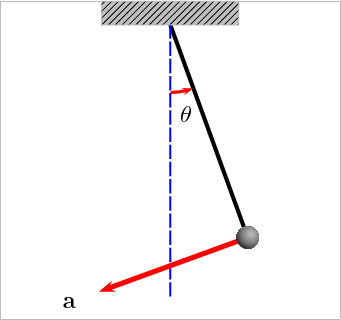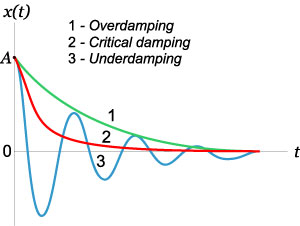Click to Chat

1800-1023-196

+91-120-4616500

CART 0

• 0

MY CART (5)

Use Coupon: CART20 and get 20% off on all online Study Material

ITEM
DETAILS
MRP
DISCOUNT
FINAL PRICE
Total Price: Rs.

There are no items in this cart.
Continue Shopping• Complete JEE Main/Advanced Course and Test Series
• OFFERED PRICE: Rs. 15,900
• View Details

Revision Notes on Oscillations

Types of Motion:-

(a) Periodic motion:- When a body or a moving particle repeats its motion along a definite path after regular intervals of time, its motion is said to be Periodic Motion and interval of time is called time or harmonic motion period (T). The path of periodic motion may be linear, circular, elliptical or any other curve.

(b) Oscillatory motion:-‘ To and Fro' type of motion is called an Oscillatory Motion. It need not be periodic and need not have fixed extreme positions.The force/torque acting in oscillatory motion (directed towards equilibrium point) is called restoring force/torque.(c) Simple harmonic motion (SHM):- Simple harmonic motion is the motion in which the restoring force is proportional to displacement from the mean position and opposes its increase.

Simple harmonic motion (SHM):- A particle is said to move in SHM, if its acceleration is proportional to the displacement and is always directed towards the mean position.

Conditions of Simple Harmonic MotionFor SHM is to occur, three conditions must be satisfied.

(a) There must be a position of stable equilibrium

At the stable equilibrium potential energy is minimum.

So, dU/dy= 0 and d2U/dy2> 0

(b) There must be no dissipation of energy

(c) The acceleration is proportional to the displacement and opposite in direction.

That is,a = -ω2y

Equation of SHM:-

(a)   F = -ky (b) d2y/dt2 +ω2y = 0

Here ω = √k/m (k is force constant)

Displacement (y) :- Displacement of a particle vibrating in SHM, at any instant , is defined as its distance from the mean position at that instant.y = r sin (ωt+?)

Here ? is the phase and r is the radius of the circle.

Condition:

When, ? = 0, then, y = r sin ωt

and

When, ? = π/2, then,y = rcosωt

Amplitude (r):-

Amplitude of a particle, vibrating in SHM, is defined as its maximum displacement on either side of mean position.

As the extreme value of value of ωt = ± 1, thus, y = ±r

Velocity (V):-V= dy/dt = rωcos(ωt+?)= vcos(ωt+?) = ω√r2-y2

Here v is the linear velocity of the particle.

Condition:- When, y = 0, then, V = v = rω

and

When, y = ±r, then, V=0

A particle vibrating in SHM, passes with maximum velocity through the mean position and is at rest at the extreme positions.

y2/r2 + y2/ω2r2 = 1

Acceleration (a): a = dV/dt = (-v2/r) sinωt = -ω2y

Condition:-

When, y = 0, then, a = 0

And,

When, y = ±r, then, a = ±ω2r

A particle vibrating in SHM, has zero acceleration while passing through mean position and has maximum acceleration while at extreme positions.

(i) Acceleration is directly proportional to y (displacement).

(ii) Acceleration is always directed towards the mean position.

Time period (T):- It is the time taken by the particle to complete one vibration.

(a) T = 2π/ω

(b) T =2π√(displacement/acceleration)

(c) T = 2π√m/k

Frequency (f):-It is the number of vibrations made by the body in one second.

(a) f=1/T

(b) f=1/2π√k/m

Angular frequency (ω):-

(a) ω = 2π/T

(b) ω =√(acceleration /displacement)

Relation betweenAngular frequency (ω) and Frequency (f):- ω = 2πf=√k/m

Phase:-

(a) Phase of a particle is defined as its state as regards its position and direction of motion.

(b) It is measured by the fraction of time period that has elapsed since the particle crossed its mean position, last, in the positive direction.

(c) Phase can also be measured in terms of the angle, expressed as a fraction of 2π radian, traversed by the radius vector of the circle of reference while the initial position of the radius vector is taken to be that which corresponds to the instant when the particle in SHM is about to cross mean position in positive direction.

Energy in SHM:-

(a) Kinetic Energy (Ek):-

Ek = ½ mω2(r2-y2) = ½ mω2r2cos2ωt

When, y = 0, then, (Ek)max = ½ mω2r2    (maximum)

And

When, y = ±r, then, (Ek)min =0    (minimum)

(b) Potential Energy (Ep):-

Ep = ½ mω2r2 = ½ mω2r2sin2ωt

(Ep)max = ½ mω2r2

(c) Total Energy (E):-

E = Ek +Ep=½ mω2r2 = consereved

E = (Ek)max =(Ep)max

Average Kinetic Energy:-<Ek> = (¼) mω2r2

Average Potential Energy:-<Ep> = (¼) mω2r2

<E/2> = <Ek> = <Ep>

Spring-mass system:-

(a) mg=kx0

(b) Time period, T = 2π√m/k = 2π√x0/g

Massive spring:-Time period, T = 2π√[m+(ms/3)]/k

Cutting a spring:-

(a) Time period, T'= T0/√n

(b) Frequency,f' =√(n) f0

(c) Spring constant,k' =nk

(d) If spring is cut into two pieces of length l1 and l2 such that, l1= nl2, then,

k1 = (n+1/n)k,

k2 = (n+1)k

and

k1l1 = k2l2

Spring in parallel connection:-?(a) Total  spring constant, k =k1+k2

(b) Time period, T = 2π√[m/(k1+k2)]

(c) If T1 = 2π√m/k1 and T2 = 2π√m/k2, then,

T= T1T2/√ T12+ T12 and ω2=ω12+ω22

Spring in series connection:-(a) Total  spring constant, 1/k = 1/k1+1/k2 or, k = k1k2/ k1+k2

(b) Time period, T2 =T12+ T22

(c) T = 2π√[m(k1+k2)/k1k2]

(d) 1/ω2= 1/ω12+1/ω22

(e) f = 1/2π √[k1k2/m(k1+k2)]

Law’s of simple pendulum:-?Laws of isochronisms:- Its states that (≤4°), the time period of a simple pendulum is independent of its amplitude.

Laws of length:- It states that time period of a simple pendulum varies directly as the square root of its length.

T∝√l

Law of acceleration due to gravity:- It states that, the time period of a simple pendulum varies inversely as the square root of acceleration due to gravity at that place.

T∝1/√g

So, Time period of simple pendulum, T = 2π√l/g(a) When placed inside a lift being accelerated upwards, the effective value of g increases. Thus the time period of pendulum decreases.

(b) When placed inside a lift being accelerated downwards, the effective value of g decreases. Thus the time period of pendulum increases.

(c) Time period of the pendulum increases at higher altitudes due to decrease in g.

(d) Time period of the pendulum at a place below the surface of earth decrease due to increase in g.(e) At the center of earth (g=0). So the time period is infinite.

(f) Time period is greater at equator than at poles.

(g) Due to decrease in the value of g due to rotation of earth, the time period of the pendulum increases as the earth rotates about its axis.

(h) Equation of motion:-d2θ/dt2+(g/l)θ = 0

(i)  Frequency, f =1/2π √(g/l)

(j) Angular frequency, ω =√(g/l)

Second Pendulum:-A second’spendulumis thatpendulum whose time perios is two second.

(a) T = 2 sec

(b) l = 0.9925 m

Mass-less loaded spring in the horizontal alignment:-

Force, F = -kx

Acceleration, a =-kx/m

Time period, T = 2π√m/k

Frequency,f = 1/2π√k/m

Time period of mass-less loaded spring in the vertical alignment:-

T = 2π√m/k  and T = 2π√l/g

Time period of bar pendulum:-T = 2π√I/mgl

Here I is the rotational inertia of the pendulum.

and

T = 2π√L/g

Here, L = (k2/l)+l

Time period of torsion pendulum:-(a) T=2π√I/C

Here I is therotational inertia of the pendulum and C is the restoring couple per unit angular twist.

(b) Equation of motion:-d2θ/dt2+(C/I)θ = 0

Here, θ =θ0 sin (ωt+?)

(c) Angular frequency, ω = √C/I

(d) Frequency, f = 1/2π√C/I

Conical Pendulum:-Time period, T = 2π√(Lcosθ/g)

Velocity, v = √(gRtanθ)

Restoring couple (τ):-

?=Cθ

Here C is the restoring couple per unit angular twist and θ is the twist produced in the wire.

Liquid contained in a U-tube:-

Time period, T =2π√l/g

Electrical oscillating circuit:-

Time period, T =2π√LC

Here, L is the inductance and C is the capacitance.

Angular frequency, ω = 1/√LC

Ball in a bowl:-

Time period, T = 2π√[(R-r)/g]

Free vibrations:- Vibrationsof a body are termed as free vibrations if it vibrates in the absence of any constraint.

Damped Vibrations:-?Equation:d2y/dt2 + 2µdy/dt+ω2y = 0

Here amplitude, R = Ae-µt

And

ω' = √ω2-µ2

(a)   µ<<ω signifies the body will show oscillatory behavior with gradually decreasing amplitude.

(b)   µ>>ω signifies the amplitude may decrease from maximum to zero without showing the oscillatory behavior.

(c)    In between the above two cases, the body is in the state of critically damped.

(d)   Time period of oscillation, T' = 2π/ω' = 2π/√ω2-µ2. Thus, presence of damping factor µ in the denominator indicates an increase of time period due to damping.

Forced vibrations:- Forced vibrations is the phenomenon of setting a body into vibrations by a strong periodic force whose frequency is different from natural frequency of body.

Equation: d2y/dt2+2µdy/dt+ω2y = (F0/m) cospt

Here,µ = r/2m and ω=√k/m

Solution: y =Acos [pt-?]

Amplitude:- A = F0/m√4µ2p2+(p2-ω2)2 and Amax = F0/2µm√ω2-µ2

This state of forced vibrations in which the amplitude reaches a maximum value is known as amplitude resonance.

Amplitude vibration depends upon value of ω = √k/m. Greater the value of stiffness (k), smaller is the amplitude.

Resonance:- Resonance is the phenomenon of setting a body into vibrations by a strong periodic force whose frequency coincides with the natural frequency of the body.### Course Features

• 728 Video Lectures
• Revision Notes
• Previous Year Papers
• Mind Map
• Study Planner
• NCERT Solutions
• Discussion Forum
• Test paper with Video Solution